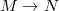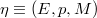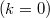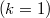ď»ż

### Introduzione

#### Abstract

En
The jet spaces of maps$M \rightarrow N$ and of the sections of a bundle$\eta \equiv (E,p,M)$ are considered,analysing their vector and affine structures. An intrinsic characterization of second order jet spaces is given. When the bundle Î· is affine or linear,further results are given. Some interesting maps concerning jet spaces are defined. The k-Lie derivable bundles are introduced and the k-Lie derivative is defined: particular cases are connections$(k=0)$, usual Lie-derivaties$(k=1)$ and Lie-derivaties of geometrical objects. Connections on a bundle are analysed and related with the affine structures of jet spaces and tangent spaces.

DOI Code: §

Full Text: PDF